Spark SQL date/time Arithmetic examples: Adding, Subtracting, etc

# Spark SQL date/time Arithmetic examples: Adding, Subtracting, etc

Last updated:

Spark 2.4.8 used

See all examples on this jupyter notebook

## Summary

Method Description
date_add(col, num_days) and date_sub(col, num_days) Add or subtract a number of days from the given date/timestamp.
Works on Dates, Timestamps and valid date/time Strings.
When used with Timestamps, the time portion is ignored
Example
datediff(col_before, col_after) Returns the number of days between two datetime columns.
Works on Dates, Timestamps and valid date/time Strings.
When used with Timestamps, the time portion is ignored.
Example
unix_timestamp(date_col) and subtract Returns the number of seconds between two date/time columns.
Works on Dates, Timestamps and valid date/time Strings.
Example
Cast to double, subtract, multiply by 1000 and cast back to long Returns the number of milliseconds between two Timestamp columns.
Works on Timestamps only.
Example
expr("INTERVAL...") Performs a precise subtraction or addition to a Timestamp, without ignoring the time portion.
Works on Dates, Timestamps and valid date/time Strings.
Example

HEADS-UP Time information is ignored. Timestamps are truncated to the day.

Use date_add(source_column, num_days) or date_sub(source_column, num_days)

Can be used on Date, Timestamp and String columns (when string is a valid date/timestamp string)

import java.sql.Timestamp

val df = Seq(
("notebook",    Timestamp.valueOf("2019-01-29 12:00:00")),
("notebook",    Timestamp.valueOf("2019-01-01 00:00:00")),
("small_phone", Timestamp.valueOf("2019-01-15 23:00:00")),
("small_phone", Timestamp.valueOf("2019-01-01 09:00:00"))
).toDF("device", "purchase_time").sort("device","purchase_time")

df.withColumn("plus_2_days",date_add($"purchase_time",2))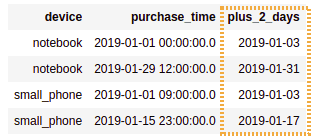Created a column by adding 2 days to column purchase_time. Time information is lost in the process, and the created column is always of time Date ## Datediff example HEADS-UP Time information is ignored. Timestamps are truncated to the day. Returns the number of days between two dates. Template: datediff($"column_AFTER", $"column_BEFORE") import java.sql.Date import org.apache.spark.sql.functions.datediff val df = Seq( ("notebook", Date.valueOf("2019-01-29"), Date.valueOf("2019-02-10")), ("notebook", Date.valueOf("2019-01-01"), Date.valueOf("2019-01-15")), ("small_phone", Date.valueOf("2019-01-15"), Date.valueOf("2019-01-05")), ("small_phone", Date.valueOf("2019-01-01"), Date.valueOf("2019-01-20")) ).toDF("device", "purchase_date", "arrival_date").sort("device","purchase_date") df.withColumn("days_to_arrive",datediff($"arrival_date", $"purchase_date"))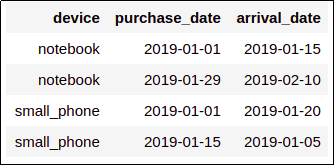Source dataframe: one column indicating when a product was purchased and another one with the date it arrived at the buyer's house.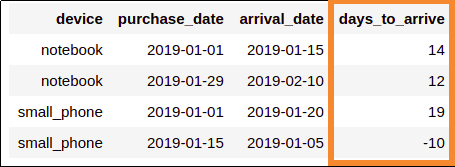Using datediff to calculate the number of days it took for a package to arrive. Negative values here probably indicate a bug somewhere in the source system code ## Difference in days Just use datediff: Datediff example ## Difference in seconds • First convert datetime to unix timestamp using unix_timestamp(datetime_col) • Then subtract import java.sql.Timestamp.valueOf import org.apache.spark.sql.functions.unix_timestamp val df = Seq( ("foo", valueOf("2019-01-01 00:00:00"), valueOf("2019-01-01 01:00:00")), // 1 hour apart ("bar", valueOf("2019-01-01 00:00:00"), valueOf("2019-01-02 00:00:00")), // 24 hours apart ("baz", valueOf("2019-01-01 00:00:00"), valueOf("2019-01-07 00:00:00")) // 7 days apart ).toDF("col1", "purchase_time", "arrival_time").sort("col1", "purchase_time") df .withColumn("diff_in_seconds", unix_timestamp($"arrival_time") - unix_timestamp($"purchase_time"))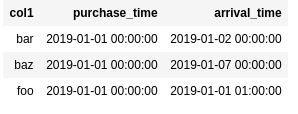BEFORE: a dataframe with two Timestamp column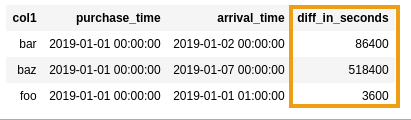AFTER: difference in seconds between purchase_time and arrival_time ## Difference in milliseconds • 1) Cast Timestamp to double • 2) Subtract both values • 3) Multiply by 1000 • 4) Cast back to long import java.sql.Timestamp.valueOf import org.apache.spark.sql.functions.to_timestamp // building the sample dataframe val df = Seq( ("foo", valueOf("2019-01-01 00:00:00.000"), valueOf("2019-01-01 00:00:00.400")), ("bar", valueOf("2019-01-01 00:00:00.000"), valueOf("2019-01-01 00:00:00.650")), ("baz", valueOf("2019-01-01 00:00:00.000"), valueOf("2019-01-01 00:01:00.000")) ).toDF("col1", "time_before", "time_after") // cast to double, subtract, multiply by 1000, cast back to long df .withColumn("diff_millis",$"time_after".cast("double") - $"time_before".cast("double")) .withColumn("diff_millis", ($"diff_millis"*1000).cast("long"))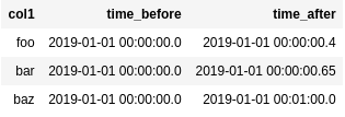BEFORE: source dataframe has two timestamp
columns with microseconds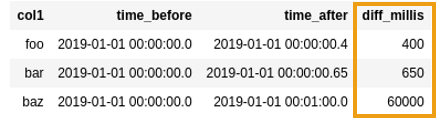AFTER: created a new column with the
difference between the two timestamp
columns (in milliseconds)

## Difference in hours

• Convert to seconds with cast("double")
• Subtract
• Divide by 36001
import java.sql.Timestamp.valueOf
import org.apache.spark.sql.functions.expr

val df = Seq(
("foo", valueOf("2019-10-10 00:00:00.000"), valueOf("2019-10-10 01:00:00.000")), // exactly 1 hour
("bar", valueOf("2019-10-10 00:00:00.000"), valueOf("2019-10-10 01:00:00.123")), // one hour and some millis
("baz", valueOf("2019-10-10 00:00:00.000"), valueOf("2019-10-11 01:30:00.123")) // one day and one and a half hours
).toDF("col1", "purchase_time", "arrival_time")

val seconds_in_hour = 60*60

df
.withColumn("difference_in_seconds", $"arrival_time".cast("double") -$"purchase_time".cast("double"))
.withColumn("difference_in_hours", $"difference_in_seconds" / seconds_in_hour) .select("col1", "purchase_time", "arrival_time", "difference_in_hours")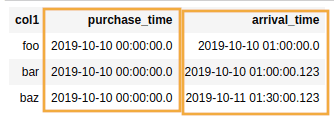The time a given item was purchased and the time it arrived at the client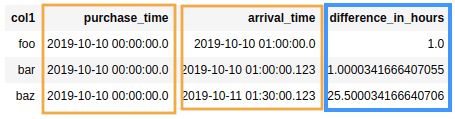Note that the result is a float, to signal when the difference in times is not a full hour. ## Expr INTERVAL example If you use date_sub (or date_add) on timestamps, they get truncated to the date at zero hours. To avoid truncation (i.e. to subtract a precise period) use $"colname" - expr("INTERVAL ...").

Example: subtract 24 hours from timestamp:

import java.sql.Timestamp
import org.apache.spark.sql.functions.expr

val df = Seq(
("foo", Timestamp.valueOf("2019-10-10 00:45:00")),
("bar", Timestamp.valueOf("2019-10-10 12:34:56")),
("baz", Timestamp.valueOf("2019-10-10 23:59:00"))
).toDF("col1", "timestamp_col")

// naturally you can also use "+" for adding periods
.withColumn("timestamp_minus_24_hours", $"timestamp_col" - expr("INTERVAL 24 HOURS"))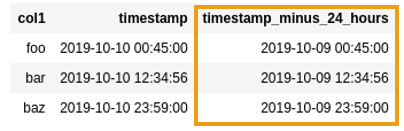To subtract 24 hours from a timestamp column use $"col" - expr("INTERVAL 24 HOURS")